# Solutions Notes for Class 12 Chemistry

0
880

CBSE Class 12 Chemistry
Quick Revision Notes
Chapter 2
Solutions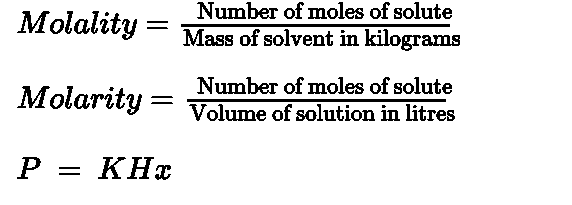The difference in boiling points of solution Tb and pure solvent T® is called elevation in boiling point• Solutions: Solutions are the homogeneous mixtures of two or more than two components.
• Binary solution: A solution having two components is called a binary solution.
• Components of a binary solution.

It includes solute and solvent.

1. When the solvent is in solid state, solution is called solid solution.
2. When the solvent is in liquid state, solution is called liquid solution.
3. When the solvent is in gaseous state, solution is called gaseous solution.
• Concentration: It is the amount of solute in given amount of solution.
• Mass by volume percentage (w/v): Mass of the solute dissolved in 100 mL of solution.
• Molality (m) is the number of moles of solute present in 1kg of solvent.
• Molarity (M) is the number of moles of solute present in 1L of solution.
• Normality is the number of gram equivalent of solute dissolved per litre of solution.
• Solubility: It is the maximum amount that can be dissolved in a specified amount of solvent at a specified temperature.
• Saturated solution: It is a solution in which no more solute can be dissolved at the same temperature and pressure.
• In a nearly saturated solution if dissolution process is an endothermic process, solubility increases with increase in temperature.
• In a nearly saturated solution if dissolution process is an exothermic process, solubility decreases with increase in temperature.
• Henry’s Law: It states “at a constant temperature the solubility of gas in a liquid is directly proportional to the pressure of gas”. In other words, “the partial pressure of gas in vapour phase is proportional to the mole fraction of the gas in the solution”.
• When a non-volatile solute is dissolved in a volatile solvent, the vapour pressure of solution is less than that of pure solvent.
• Raoult’s law: It states that “for a solution of volatile liquids the partial vapour pressure of each component in the solution is directly proportional to its mole fraction”.
• Using Dalton’s law of partial pressure the total pressure of solution is calculated.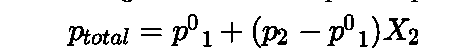• Comparison of Raoult’ law and Henry’s law: It is observed that the partial pressure of volatile component or gas is directly proportional to its mole fraction in solution. In case of Henry’s Law the proportionality constant is KH and it is different from p10 which is partial pressure of pure component. Raoult’s Law becomes a special case of Henry’s Law when KH becomes equal to p10 in Henry’s law.
• Classification of liquid-liquid solutions: It can be classified into ideal and non-ideal solutions on basis of Raoult’s Law.

• Ideal solutions:

1. The solutions that obey Raoult’s Law over the entire range of concentrations are known as ideal solutions.

3. The intermolecular attractive forces between solute molecules and solvent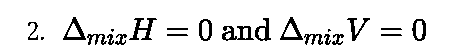molecules are nearly equal to those present between solute and solvent molecules i.e. A-A and B-B interactions are nearly equal to those between A-B.

Non-ideal solutions:

1. When a solution does not obey Raoult’s Law over the entire range of concentration, then it is called non-ideal solution.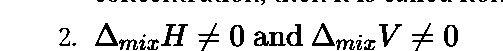3. The intermolecular attractive forces between solute molecules and solvent

molecules are not equal to those present between solute and solvent molecules i.e. A-A and B-B interactions are not equal to those between A-B

• Types of non- ideal solutions:
1. Non ideal solution showing positive deviation
2. Non ideal solution showing negative deviation
• Non ideal solution showing positive deviation
1. The vapour pressure of a solution is higher than that predicted by Raoult’s Law.
2. The intermolecular attractive forces between solute-solvent molecules are weaker than those between solute-solute and solvent-solvent molecules i.e., A-B < A-A and B-B interactions.
• Non ideal solution showing negative deviation
1. The vapour pressure of a solution is lower than that predicted by Raoult’s Law.
2. The intermolecular attractive forces between solute-solvent molecules are stronger than those between solute-solute and solvent-solvent molecules i.e. A-B > A-A and B-B interactions.
• Azeotopes: These are binary mixtures having same composition in liquid and vapour

phase and boil at constant temperature. Liquids forming azeotrope cannot be

separated by fractional distillation.

• Types of azeotropes: There are two types of azeotropes namely,
1. Minimum boiling azeotrope
2. Maximum boiling azeotrope

• The solutions which show a large positive deviation from Raoult’s law form minimum

boiling azeotrope at a specific composition.

• The solutions that show large negative deviation from Raoult’s law form maximum

boiling azeotrope at a specific composition.

• Colligative properties: The properties of solution which depends on only the number of solute particles but not on the nature of solute are called colligative properties.
• Types of colligative properties: There are four colligative properties namely,
1. Relative lowering of vapour pressure
2. Elevation of boiling point
3. Depression of freezing point
4. Osmotic pressure
• Relative lowering of vapour pressure: The difference in the vapour pressure of pure solvent p\j and solution pi represents lowering in vapour pressure(p® —pi).
• Relative lowering of vapour pressure: Dividing lowering in vapour pressure by vapour pressure of pure solvent is called relative lowering of vapour pressure• Relative lowering of vapour pressure is directly proportional to mole fraction of solute. Hence it is a colligative property.• For a dilute solution elevation of boiling point is directly proportional to molal concentration of the solute in solution. Hence it is a colligative property.• Depression of freezing point: The lowering of vapour pressure ofsolution causes a lowering of freezing point compared to that of pure solvent.The difference in freezing point of the pure solvent T® and solution Tf is called the depression in freezing point.

passage of solvent into solution through a semipermeable membrane is called osmotic pressure.

• Osmotic pressure is a colligative property as it depends on the number of solute particles and not on their identity.
• For a dilute solution, osmotic pressure (7r) is directly proportional to the molarity (C) of the solution i.e. 7r= CRT
• Osmotic pressurecan also be used to determine the molar mass of solute using the equatioi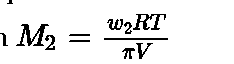• Isotonic solution: Two solutions having same osmotic pressure at a given temperature are called isotonic solution.
• Hypertonic solution: If a solution has more osmotic pressure than other solution it is called hypertonic solution.
• Hypotonic solution: If a solution has less osmotic pressure than other solution it is called hypotonic solution.
• Reverse osmosis: The process of movement of solvent through a semipermeable membrane from the solution to the pure solvent by applyingexcess pressure on the solution side is called reverse osmosis.
• Colligative properties help in calculation of molar mass of solutes.
• Abnormal molar mass: Molar mass that is either lower or higher than expected or normalmolar mass is called as abnormal molar mass.
• Van’t Hoff factor: Van’t Hoff factor (i)accounts for the extent of dissociation or association.• For a dilute solution depression in freezing point is a colligative property because it is

directly proportional to molal concentration of solute.

• Osmosis: The phenomenon of flow of solvent molecules through a semi permeable membrane from pure solvent to solution is called osmosis.
• Osmotic pressure: The excess pressure that must be applied to solution to prevent the
• Value of i is less than unity in case solute undergo association and the value of i is greater than unity in case solute undergo dissociation.
1. Inclusion of van’t Hoff factor modifies the equations for colligative properties as: